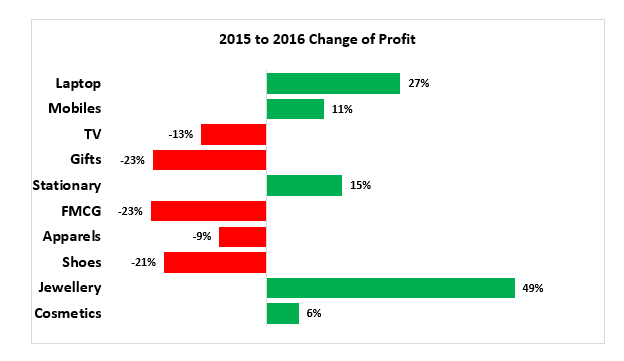# Introduction – Variance Analysis

Variance analysis refers to a difference between two values. The deviation may favorable or unfavorable as under:Variance is generally calculated as per below parametersThe time period for measuring deviation can be as under:

• Current Month vs Previous Month
• Current Year vs Previous Year
• First Quarter vs Second Quarter

Variance Reports are made to find out the % increase or decrease for Profit, Revenue, Cost Expenses, Attrition, Manpower Count, Salary, Recruitment Cost etc.

Examples of Variance Analysis charts